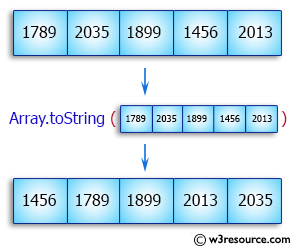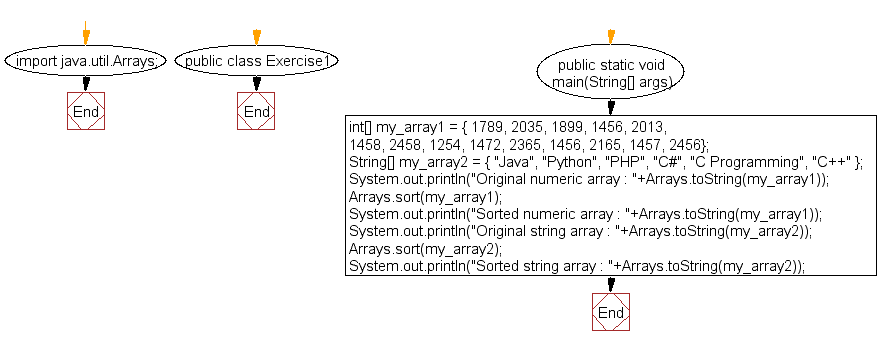﻿ Java exercises: Sort a numeric array and a string array - w3resource# Java Array Exercises: Sort a numeric array and a string array

## Java Array: Exercise-1 with Solution

Write a Java program to sort a numeric array and a string array.

Pictorial Presentation:Sample Solution:

Java Code:

``````import java.util.Arrays;
public class Exercise1 {
public static void main(String[] args){

int[] my_array1 = {
1789, 2035, 1899, 1456, 2013,
1458, 2458, 1254, 1472, 2365,
1456, 2165, 1457, 2456};

String[] my_array2 = {
"Java",

"Python",
"PHP",
"C#",
"C Programming",
"C++"
};
System.out.println("Original numeric array : "+Arrays.toString(my_array1));
Arrays.sort(my_array1);
System.out.println("Sorted numeric array : "+Arrays.toString(my_array1));

System.out.println("Original string array : "+Arrays.toString(my_array2));
Arrays.sort(my_array2);
System.out.println("Sorted string array : "+Arrays.toString(my_array2));
}
}
```
```

Sample Output:

```Original numeric array : [1789, 2035, 1899, 1456, 2013, 1458, 2458, 1254, 1472, 2365, 1456, 2165, 1457, 2456]
Sorted numeric array : [1254, 1456, 1456, 1457, 1458, 1472, 1789, 1899, 2013, 2035, 2165, 2365, 2456, 2458]
Original string array : [Java, Python, PHP, C#, C Programming, C++]
Sorted string array : [C Programming, C#, C++, Java, PHP, Python]
```

Flowchart:Visualize Java code execution (Python Tutor):

Java Code Editor:

Improve this sample solution and post your code through Disqus

What is the difficulty level of this exercise?

Test your Programming skills with w3resource's quiz.

﻿

## Java: Tips of the Day

countOccurrences

Counts the occurrences of a value in an array.

Use Arrays.stream().filter().count() to count total number of values that equals the specified value.

```public static long countOccurrences(int[] numbers, int value) {
return Arrays.stream(numbers)
.filter(number -> number == value)
.count();
}
```

Ref: https://bit.ly/3kCAgLb